# 10个强大的Python数据科学技巧

2020/09/23 19:20

### 介绍

Python不仅限于Pandas，NumPy和scikit-learn（尽管它们在数据科学中绝对必不可少）！我们可以使用大量的Python技巧来改进代码，加速数据科学任务并提高编写代码的效率。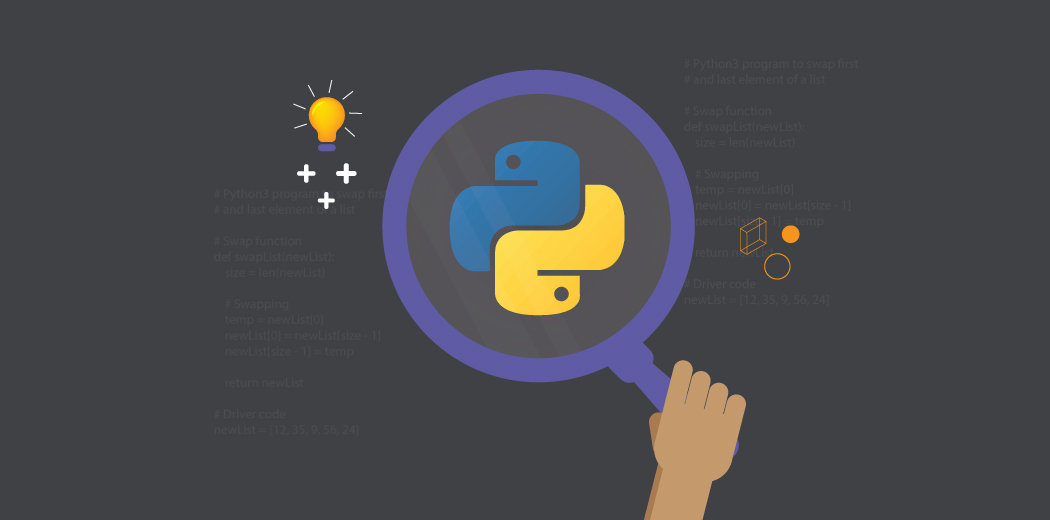Python和数据科学世界的新手？这是一门精妙而全面的课程，可帮助你同时入门：

#### 1. zip：在Python中合并多个列表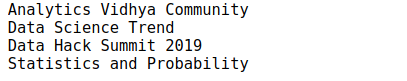!pip3 install gmplot


import pandas as pd
import gmplot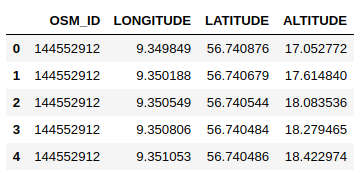# latitude and longitude list
latitude_list = data['LATITUDE']
longitude_list = data['LONGITUDE']

# center co-ordinates of the map

# plot the co-ordinates on the google map
gmap.scatter( latitude_list, longitude_list, '# FF0000', size = 40, marker = True)

gmap.heatmap(latitude_list, longitude_list)

gmap.draw( "mymap.html" )


#### 3. category_encoders：使用15种不同的编码方案对分类变量进行编码

!pip3 install category-encoders



import pandas as pd
import category_encoders as ce

# create a Dataframe
data = pd.DataFrame({ 'gender' : ['Male', 'Female', 'Male', 'Female', 'Female'],
'class' : ['A','B','C','D','A'],
'city' : ['Delhi','Gurugram','Delhi','Delhi','Gurugram'] })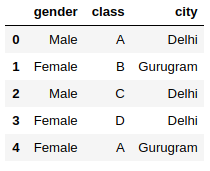# One Hot Encoding
# create an object of the One Hot Encoder

ce_OHE = ce.OneHotEncoder(cols=['gender','city'])

# transform the data
data = ce_OHE.fit_transform(data)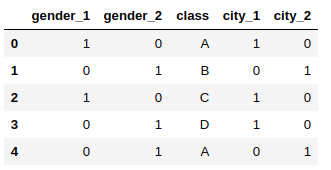category_encoders支持大约15种不同的编码方法，例如：

• 哈希编码
• LeaveOneOut编码
• 顺序编码
• 二进制编码
• 目标编码

#### 4. progress_apply：监视你花费在数据科学任务上的时间

import pandas as pd
from tqdm._tqdm_notebook import tqdm_notebook
from pysal.lib.cg import harcdist
tqdm_notebook.pandas()# calculate the distance of each data point from # (Latitude, Longitude) = (58.4442, 9.3722)

def calculate_distance(x):
return harcdist((x['LATITUDE'],x['LONGITUDE']),(58.4442, 9.3722))

data['DISTANCE'] = data.progress_apply(calculate_distance,axis=1)


#### 5. pandas_profiling：生成数据集的详细报告

pandas_profiling 是一个Python软件包，可减少执行初始数据分析步骤所需的大量工作。该软件包只需一行代码即可生成有关我们数据的详细报告！

import pandas as pd
import pandas_profiling

pandas_profiling.ProfileReport(data)


• 警告，例如： Item_Identifier具有高基数：1559个不同的值警告
• 所有类别变量的频率计数
• 数字变量的分位数和描述统计
• 相关图

#### 6. grouper：对时间序列数据进行分组

import pandas as pd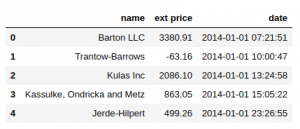data['date'] = pd.to_datetime(data['date'])


data.set_index('date').groupby('name')["ext price"].resample("M").sum()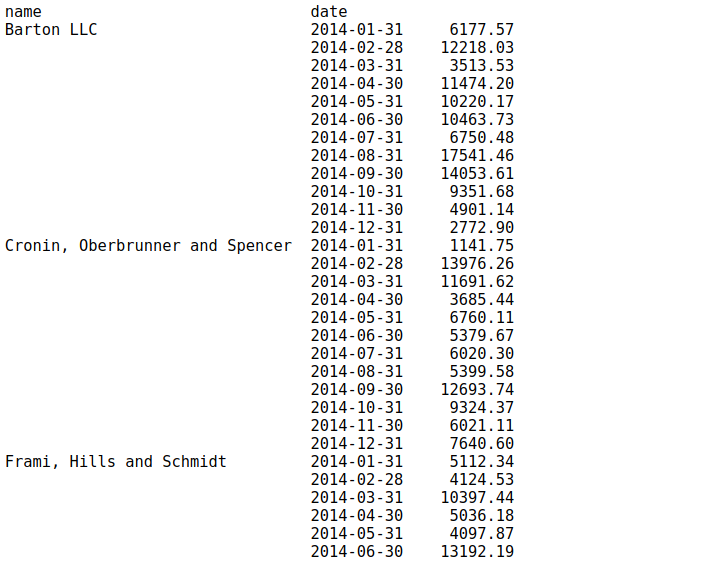data.groupby(['name', pd.Grouper(key='date', freq='M')])['ext price'].sum()#### 7. unstack：将索引转换为Dataframe的列

data.groupby(['name', pd.Grouper(key='date', freq='M')])['ext price'].sum().unstack()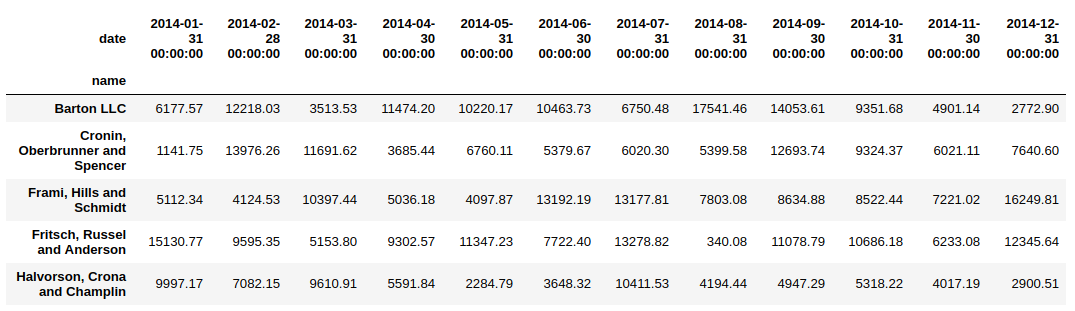非常有用！注意：如果索引不是MultiIndex，则输出将是Series。

#### 8.％matplotlib Notebook：Jupyter Notebook中的交互式绘图

%matplotlib notebook
import matplotlib.pyplot as plt

# scatter plot of some data # try this on your dataset
plt.scatter(data['quantity'],data['unit price'])


#### 9. %% time：检查特定Python代码块的运行时间

%%time
def myfunction(x) :
for i in range(1,100000,1) :
i=i+1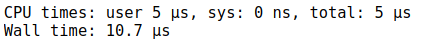#### 10：rpy2：R和Python在同一个Jupyter Notebook中！

R和Python是数据科学世界中最好的和最受欢迎的两种开源编程语言。R主要用于统计分析，而Python提供了一个简单的接口，可将数学解决方案转换为代码。

!pip3 install rpy2


%load_ext rpy2.ipython
%R require(ggplot2)

import pandas as pd
df = pd.DataFrame({
'Class': ['A', 'A', 'A', 'V', 'V', 'A', 'A', 'A'],
'X': [4, 3, 5, 2, 1, 7, 7, 5],
'Y': [0, 4, 3, 6, 7, 10, 11, 9],
'Z': [1, 2, 3, 1, 2, 3, 1, 2]
})

%%R -i df
ggplot(data = df) + geom_point(aes(x = X, y= Y, color = Class, size = Z))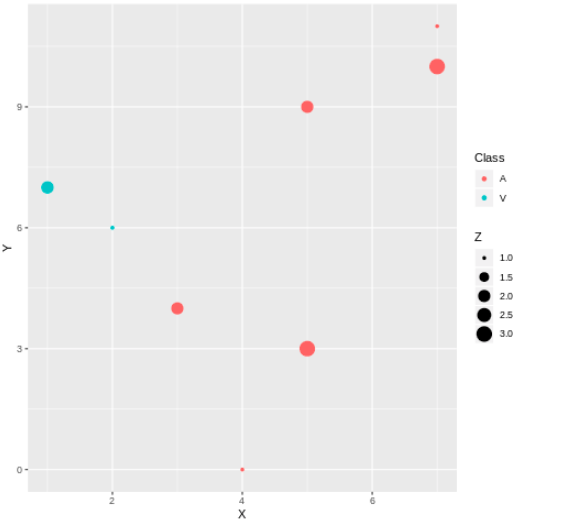### 尾注

sklearn机器学习中文官方文档： http://sklearn123.com/

2
3 收藏

### 作者的其它热门文章0 评论
3 收藏
2